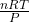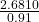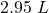## What volume is occupied by 0.104 mol of helium gas at a pressure of 0.91 atm and a temperature of 314 K ?

Question

What volume is occupied by 0.104 mol of helium gas at a pressure of 0.91 atm and a temperature of 314 K ?

in progress 0
2 months 2021-07-12T02:33:16+00:00 1 Answers 2 views 0

The volume will be “2.95 L“.

Explanation:

Given:

n = 0.104

p = 0.91 atm

T = 314 K

Now,

The Volume (V) will be:

=By putting the values, we get

===